﻿ Objects > Functions > Using Functions

# Using FunctionsOnce you've defined a function, you can use it in many ways: you can plot it, use it in a calculation or to define another function, edit it, differentiate it, trace its family, construct its family, transform it symbolically, transform its plot with a custom transformation, use it to define a composite function or a parametric plot, or create a sound button.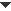Plot a Function

To define a new function and plot it immediately on the marked coordinate system, choose Graph | Plot New Function to open Sketchpad’s Calculator. Use it to define the function. When you close the Calculator, the function is plotted in the form that was set in the Calculator’s Equation pop-up menu. The function plot can take any of four possible forms.

 y = f(x) This is the default form. x = f(y) Use this form to plot the y = f(x) function with the axes reversed. r = f(θ) You’ll normally use the last two forms with a polar grid. θ = f(r) Use this form to graph θ as a function of r with a polar grid.

To plot one or more existing functions on the marked coordinate system, select every function you want to plot and choose Each function is plotted in the form that was set in the  Calculator’s Equation pop-up menu at the time you defined that function.Use a Function in a Calculation or Function Definition
 Once you’ve defined a function, you can use it in later calculations and function definitions. To use an existing function in a calculation or in a new function definition, click the function in the sketch to enter it into the Calculator. If the Calculator is hiding the function in the sketch, you may have to move the Calculator out of the way first. You can also enter functions from the Function pop-up menu in the Calculator. This menu includes many predefined functions, along with every selected user-defined function in your sketch.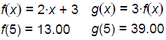Edit a Symbolic Function
 You can edit a symbolically defined function to change its definition or how it’s plotted. Editing a function is useful, for example, if you’ve plotted the graph of y = 2·sin(x), and you want to change the function to y = 3·sin(x) in order to see how the two graphs differ. Instead of changing the constant to 3, you can insert a new parameter, allowing you to investigate the plots of the family of functions y = a·sin(x). To edit a function, select that function, choose and use the Calculator to change the definition. You can also right-click (Win) or Ctrl-click (Mac) the function and choose Edit Function from the Context menu. When you edit a function, you can redefine it in any way you want — introducing new parameters and existing parameters, measurements and calculations — provided you don’t create a circular definition. (A circular definition is one that uses a calculation that depends on the function you’re editing. For instance, if you’ve defined a function f and used it to calculate f(3) in your sketch, you cannot later edit function f so that it uses the calculation of f(3) in its definition.) Choose a new equation form while editing a function to change how that function is plotted. For instance, to plot a function f as a polar function, use the Equation pop-up menu to change the form from y = f(x) to r = f(θ).Differentiate a Function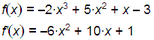To create the derivative of a function with respect to its independent variable, select the function, then choose The result is a derivative function that can be plotted or evaluated like any other function.Trace a Family of Functions
 Use parameters or sliders in a function definition to investigate families of functions. After you define the function and plot its graph, you can change or animate the parameter or slider, and observe or trace the family of functions. While you’re using the function Calculator to enter or edit a function, you can create a new parameter or use an existing parameter or other measurement from your sketch. When the value of this parameter or measurement changes, the definition of the function changes. For example, if you create parameter a while you’re specifying the function f(x) = a·sin (x), you can investigate the behavior and plots of this entire family of functions, including functions such as f(x) = –1·sin(x) and f(x) = 3·sin (x) by varying the parameter a. Similarly, use three parameters as you define the function f(x) = a·x2 + b·x + c to investigate how changing each parameter’s value affects the function plot. With the Calculator open add a new parameter to the function definition by choosing Number | New Parameter from the Calculator’s Values pop-up menu. Insert an existing parameter by clicking on it in the sketch.Construct a Family of Functions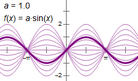When you use a parameter or slider in a function definition, you can construct the family of functions. You must first plot the function itself. Then select both the function plot and the parameter or slider used in the function definition and chooseTransform a Function Symbolically

Once you’ve defined a function f(x) — for example, f(x) = x2 — you can define and plot other functions that are transformations of f(x). Transformations of f(x) include such functions as these:

 g(x) = 2·f(x) vertical stretch h(x) = f(–x) reflection across y-axis j(x) = –3·f(2x + 5) – 1 horizontal shrink (1/2) and translate left (5), followed by vertical reflection (–), stretch, (3), and translate down (–1) k(x) = f(x – h) + k translate right by h and up by k

To define a function that is a transformation of f(x), choose Define the new function as you normally would, inserting the original function f wherever you want by clicking it in the sketch.Custom Transform a Function Plot
 Once you’ve plotted a function, you can define a custom transformation and apply the transformation to the function plot. For instance, if you define a custom transformation to do a vertical stretch, you can apply the transformation to a function plot. In this example, point B is plotted at (xA, k·yA), and points A and B are used to define a custom transformation. This custom transformation is a vertical stretch by the factor k. When the custom transformation is applied to the plot of f(x) (in blue), the result is the vertically stretched red plot. Because the vertical stretch depends on parameter k, you can use this construction to explore a variety of vertical stretches of the blue function plot.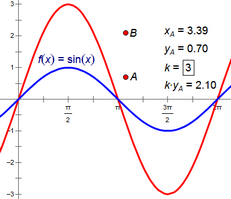Define a Composite Function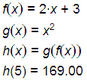Composite functions are functions of functions. For instance, h(x) = g(f(x)) is called the composition of functions g and f, and can be thought of as follows: “First evaluate function f at the input value. Then use this result as the input for function g. The result of evaluating g is the output of the composite function g(f(x)).” To compose two functions g and f, first define each function separately. Then choose Number | New Function and use the function Calculator to define the composite function h(x). Click the functions g and f in the sketch to enter them into the Calculator. If the Calculator is hiding f or g in the sketch, you may have to move the Calculator out of the way first.Define a Parametric PlotTo create a parametric plot, define one function to represent the x value of a point and a second function to represent the y value. As the independent variable changes, the set of points determined by the values of the two functions defines the parametric plot. In this example, given any value of θ, the values of the two functions x(θ) and y(θ) determine a point. To construct the parametric plot, select these two defining functions and choose The resulting plot is in the shape of an ellipse.Create a Sound ButtonTo create a Sound button, define a mathematical function to describes air pressure over time. (The volume is determined by the amplitude of the function, and the pitch is determined by its period.) Then select the function and choose Edit | Action Buttons | Sound to create the button. (You can also select two functions to play a sound in stereo.)